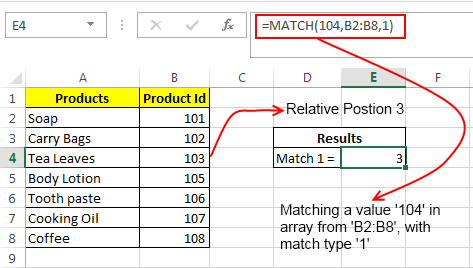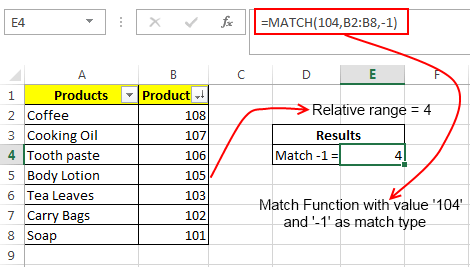# Excel Match Function – How To Use

Excel provides many formulas for finding a particular string or text in an array. One such function is MATCH, in fact Match function is designed to do a lot more than this. Today we are going to learn how to use the Excel Match function. Basically what match function does is, it scans the whole array range in order to find the specified text and thereafter it returns its position.

### Excel Match Function Definition:

Excel defines match function as: “Returns the relative position of an item in an array that matches a specified value in a specified order”. In simple plain language Match function searches for a value in a defined range and then returns its position.

### Syntax of Match Formula:

Match formula can be written as: `MATCH(lookup_value, range, match_type)`

Here: ‘lookup_value’ signifies the value to be searched in the array.

‘range’ is the array of values on which you want to perform a match.

‘match_type’ Match type is an important thing. It can have three values 1, 0 or -1.

• If ‘match_type’ has a value 1, it means that match function will find a value that is less than or equal to ‘lookup_value’. It can only be applied if the array (‘range’) is sorted in an ascending order.
• If ‘match_type’ has a value 0, it means that match function will find the first value that is equal to the ‘lookup_value’. In this case sorting of array (‘range’) is not important.
• If ‘match_type’ has a value -1, it means that match function will find the smallest value that is greater than or equal to ‘lookup_value’. It can only be applied if the array (‘range’) is sorted in a descending order.

### Few Important things about Match Formula:

1. Match is case-insensitive. It does not know the difference between upper and lower case.

2. If ‘match_type’ i.e. the third parameter of match function is omitted, then the function treats its value to be 1 as default.

3. If the Match formula cannot find any matches, it results into #N/A error.

4. Match function also supports the use of wildcard operators, but they can only be used in case of text comparisons where the ‘match_type’ is 0. We will cover this with an example later.

5. Match function does not return the matching string, it only returns the relative position of that string.

6. If the array is not sorted in the ascending order for ‘match_type’ 1 then it results into a #N/A error. Similarly #N/A error also occurs if the defined cell range is not sorted in descending order for ‘match_type’ equal to -1.

### Example of Match Formula in Excel:1. In the first example we have applied a Match function as shown in the above image.

The Match Function is applied as: `=MATCH(104,B2:B8,1)`

The Result is 3.

This means that Match searches the whole range for the value 104 but as 104 was not present in the list so it pointed to the relative position of a value slightly less than 104 i.e. 103. If in the same example the array would have the value 104 with array being sorted in ascending order then the same formula would have resulted into pointing the relative reference of 104.

2. If we apply another Match function: `=MATCH(104,B2:B8,0)` on the same data set.

Then it will result into an error as ‘0’ signifies exact match and in absence of the value 104 the function will give an error #N/A

3. If we apply another Match function: `=MATCH(104,B2:B8,-1)` on the same data set.

Then it will result into an error as the array is not sorted in descending order.4. In the second example a Match formula with match type as -1 is used.

The Result is: 4

This is because as the value 104 is not present in the array so the Match function points to the relative reference of a value slightly greater than 104 i.e. 105.

### WildCard Operators in Match Function:

Using wildcards can only prove useful in the case of exact string matches i.e. ‘match_type’ 0. Generally two types of wildcard operators can be used within the Match Function.

1. “?” Wildcard: This signifies any single character.

2. “*” Wildcard: This signifies any number of characters.In the above example we have applied a Match Formula as: `=MATCH("T?a",A2:A8,0)`

The ‘lookup_value’ contains the “?” wildcard operator which matches the array element “Tea” and hence the result of match function is the relative position of “Tea” i.e. 3

If another Match function: `=MATCH("C*e",A2:A8,0)` is applied on the same dataset. Then it will result into a value 7. As “C*e” matches “coffee” and hence the Match function gives the relative position of “Coffee” element in the array.About Ankit Kaul